# Java HashMap详解（附源码分析）

2022年3月15日
• Java核心
• 集合框架（容器）

## # 一、hash 方法的原理

static final int hash(Object key) {
int h;
return (key == null) ? 0 : (h = key.hashCode()) ^ (h >>> 16);
}


PS：读者建议范围加上 左闭右开。因为 int 类型为 4字节，也就是 32位，取值范围为 [-2^31,2^31-1]。也就是 -2147483648 到 2147483647

final V putVal(int hash, K key, V value, boolean onlyIfAbsent, boolean evict) {
HashMap.Node<K,V>[] tab; HashMap.Node<K,V> p; int n, i;
if ((tab = table) == null || (n = tab.length) == 0)
n = (tab = resize()).length;
if ((p = tab[i = (n - 1) & hash]) == null)
tab[i] = newNode(hash, key, value, null);
}


final Node<K,V> getNode(int hash, Object key) {
Node<K,V>[] tab; Node<K,V> first, e; int n; K k;
if ((tab = table) != null && (n = tab.length) > 0 &&
(first = tab[(n - 1) & hash]) != null) {}
}


a % b = a & (b-1)

a % $2^n$ = a & ($2^n$-1)

14%8，14 的二进制为 1110，8 的二进制 1000，8-1 = 7 的二进制为 0111，1110&0111=0110，也就是 0*$2^0$+1*$2^1$+1*$2^2$+0*$2^3$=0+2+4+0=6，14%8 刚好也等于 6。

a&b 操作的结果是：a、b 中对应位同时为 1，则对应结果位为 1，否则为 0

2 的整次幂刚好是偶数，偶数-1 是奇数，奇数的二进制最后一位是 1，保证了 hash &(length-1) 的最后一位可能为 0，也可能为 1（这取决于 h 的值），即 & 运算后的结果可能为偶数，也可能为奇数，这样便可以保证哈希值的均匀性。

& 操作的结果就是将哈希值的高位全部归零，只保留低位值，用来做数组下标访问。

	 10100101 11000100 00100101
&	00000000 00000000 00001111
----------------------------------
00000000 00000000 00000101


public V put(K key, V value) {
return putVal(hash(key), key, value, false, true);
}


public V get(Object key) {
HashMap.Node<K,V> e;
return (e = getNode(hash(key), key)) == null ? null : e.value;
}


static final int hash(Object key) {
int h;
return (key == null) ? 0 : (h = key.hashCode()) ^ (h >>> 16);
}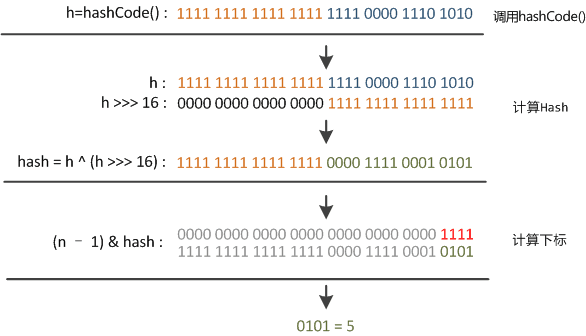## # 二、扩容机制

HashMap 的底层用的也是数组。向 HashMap 里不停地添加元素，当数组无法装载更多元素时，就需要对数组进行扩容，以便装入更多的元素。

HashMap 的扩容是通过 resize 方法来实现的，JDK 8 中融入了红黑树，比较复杂，为了便于理解，就还使用 JDK 7 的源码，搞清楚了 JDK 7 的，我们后面再详细说明 JDK 8 和 JDK 7 之间的区别。

resize 方法的源码：

// newCapacity为新的容量
void resize(int newCapacity) {
// 小数组，临时过度下
Entry[] oldTable = table;
// 扩容前的容量
int oldCapacity = oldTable.length;
// MAXIMUM_CAPACITY 为最大容量，2 的 30 次方 = 1<<30
if (oldCapacity == MAXIMUM_CAPACITY) {
// 容量调整为 Integer 的最大值 0x7fffffff（十六进制）=2 的 31 次方-1
threshold = Integer.MAX_VALUE;
return;
}

// 初始化一个新的数组（大容量）
Entry[] newTable = new Entry[newCapacity];
// 把小数组的元素转移到大数组中
transfer(newTable, initHashSeedAsNeeded(newCapacity));
// 引用新的大数组
table = newTable;
// 重新计算阈值
threshold = (int)Math.min(newCapacity * loadFactor, MAXIMUM_CAPACITY + 1);
}


a=39
b = a << 2


transfer 方法用来转移，将小数组的元素拷贝到新的数组中。

void transfer(Entry[] newTable, boolean rehash) {
// 新的容量
int newCapacity = newTable.length;
// 遍历小数组
for (Entry<K,V> e : table) {
while(null != e) {
// 拉链法，相同 key 上的不同值
Entry<K,V> next = e.next;
// 是否需要重新计算 hash
if (rehash) {
e.hash = null == e.key ? 0 : hash(e.key);
}
// 根据大数组的容量，和键的 hash 计算元素在数组中的下标
int i = indexFor(e.hash, newCapacity);

// 同一位置上的新元素被放在链表的头部
e.next = newTable[i];

// 放在新的数组上
newTable[i] = e;

// 链表上的下一个元素
e = next;
}
}
}


e.next = newTable[i]，也就是使用了单链表的头插入方式，同一位置上新元素总会被放在链表的头部位置；这样先放在一个索引上的元素终会被放到链表的尾部（如果发生了hash冲突的话），这一点和 JDK 8 有区别。

• 数组 table 的长度为 2
• 键的哈希值为 3、7、5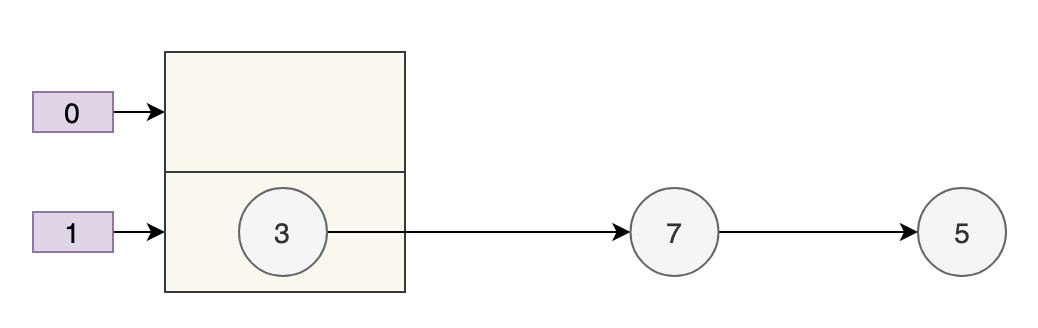• key 3 取模（3%4）后是 3，放在 table 上。
• key 7 取模（7%4）后是 3，放在 table 上的链表头部。
• key 5 取模（5%4）后是 1，放在 table 上。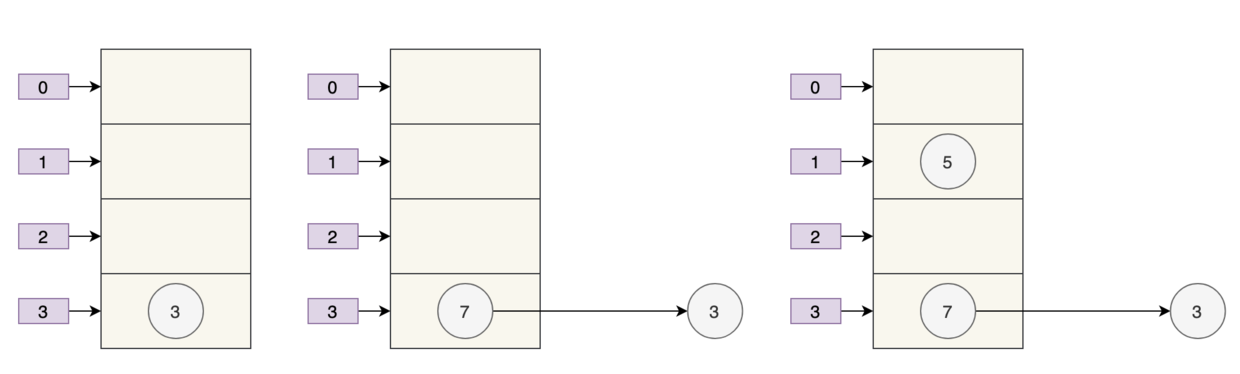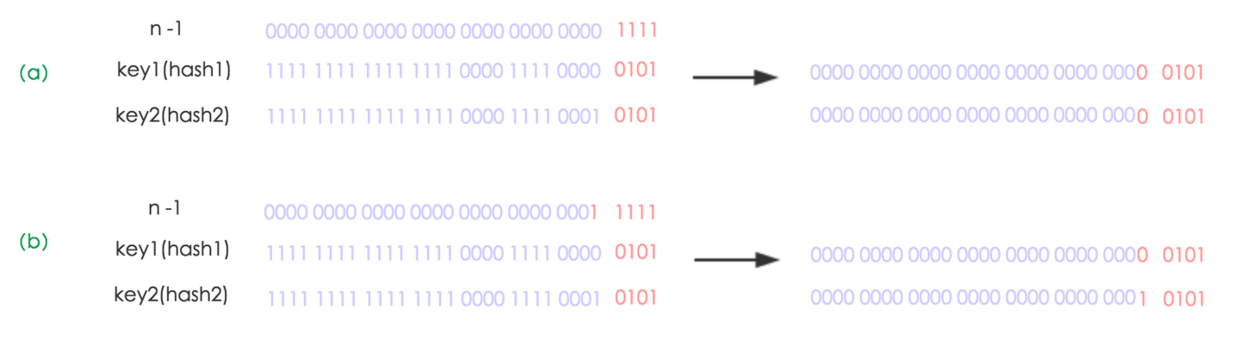n 为 table 的长度，默认值为 16。

• n-1 也就是二进制的 0000 1111（1X$2^0$+1X$2^1$+1X$2^2$+1X$2^3$=1+2+4+8=15）；
• key1 哈希值的最后 8 位为 0000 0101
• key2 哈希值的最后 8 位为 0001 0101（和 key1 不同）
• 做与运算后发生了哈希冲突，索引都在（0000 0101）上。

• n-1 也就是二进制的 0001 1111（1X$2^0$+1X$2^1$+1X$2^2$+1X$2^3$+1X$2^4$=1+2+4+8+16=31），扩容前是 0000 1111。
• key1 哈希值的低位为 0000 0101
• key2 哈希值的低位为 0001 0101（和 key1 不同）
• key1 做与运算后，索引为 0000 0101。
• key2 做与运算后，索引为 0001 0101。

• 原来的索引是 5（0 0101）
• 原来的容量是 16
• 扩容后的容量是 32
• 扩容后的索引是 21（1 0101），也就是 5+16，也就是原来的索引+原来的容量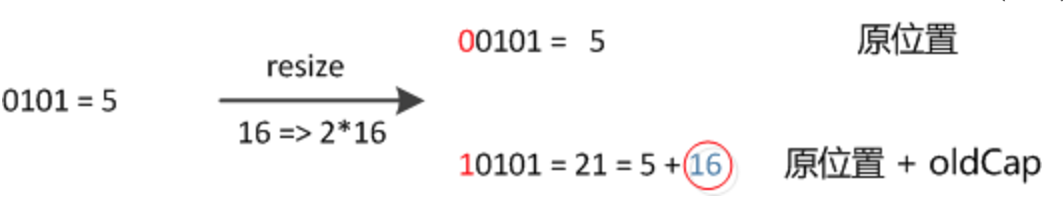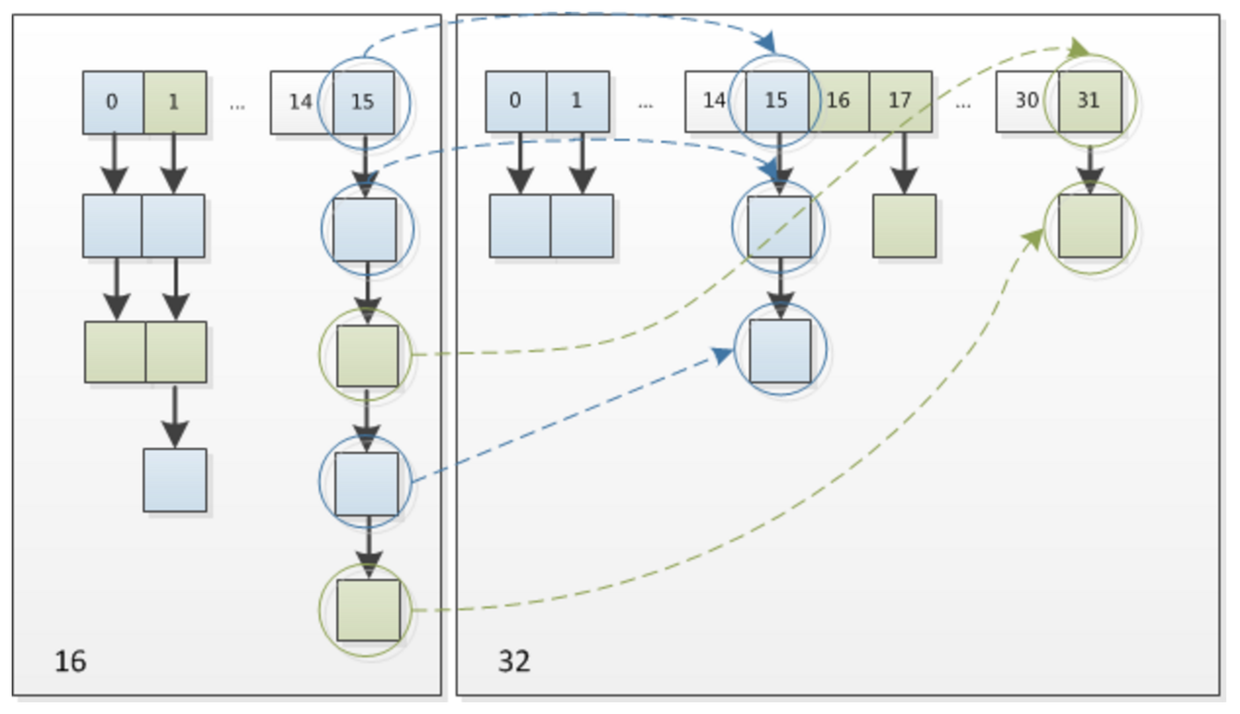JDK 8 的这个设计非常巧妙，既省去了重新计算hash的时间，同时，由于新增的1 bit是0还是1是随机的，因此扩容的过程，可以均匀地把之前的节点分散到新的位置上。

woc，只能说 HashMap 的作者 Doug Lea、Josh Bloch、Arthur van Hoff、Neal Gafter 真的强——的一笔。

JDK 8 扩容的源代码：

final Node<K,V>[] resize() {
Node<K,V>[] oldTab = table;
int oldCap = (oldTab == null) ? 0 : oldTab.length;
int oldThr = threshold;
int newCap, newThr = 0;
if (oldCap > 0) {
// 超过最大值就不再扩充了，就只好随你碰撞去吧
if (oldCap >= MAXIMUM_CAPACITY) {
threshold = Integer.MAX_VALUE;
return oldTab;
}
// 没超过最大值，就扩充为原来的2倍
else if ((newCap = oldCap << 1) < MAXIMUM_CAPACITY &&
oldCap >= DEFAULT_INITIAL_CAPACITY)
newThr = oldThr << 1; // double threshold
}
else if (oldThr > 0) // initial capacity was placed in threshold
newCap = oldThr;
else {               // zero initial threshold signifies using defaults
newCap = DEFAULT_INITIAL_CAPACITY;
}
// 计算新的resize上限
if (newThr == 0) {
float ft = (float)newCap * loadFactor;
newThr = (newCap < MAXIMUM_CAPACITY && ft < (float)MAXIMUM_CAPACITY ?
(int)ft : Integer.MAX_VALUE);
}
threshold = newThr;
@SuppressWarnings({"rawtypes","unchecked"})
Node<K,V>[] newTab = (Node<K,V>[])new Node[newCap];
table = newTab;
if (oldTab != null) {
// 小数组复制到大数组
for (int j = 0; j < oldCap; ++j) {
Node<K,V> e;
if ((e = oldTab[j]) != null) {
oldTab[j] = null;
if (e.next == null)
newTab[e.hash & (newCap - 1)] = e;
else if (e instanceof TreeNode)
((TreeNode<K,V>)e).split(this, newTab, j, oldCap);
else { // preserve order
// 链表优化重 hash 的代码块
Node<K,V> loHead = null, loTail = null;
Node<K,V> hiHead = null, hiTail = null;
Node<K,V> next;
do {
next = e.next;
if ((e.hash & oldCap) == 0) {
if (loTail == null)
else
loTail.next = e;
loTail = e;
}
else {
if (hiTail == null)
else
hiTail.next = e;
hiTail = e;
}
} while ((e = next) != null);
// 原来的索引
if (loTail != null) {
loTail.next = null;
}
// 索引+原来的容量
if (hiTail != null) {
hiTail.next = null;
}
}
}
}
}
return newTab;
}


## # 三、加载因子为什么是0.75

JDK 8 中的 HashMap 是用数组+链表+红黑树实现的，我们要想往 HashMap 中放数据或者取数据，就需要确定数据在数组中的下标。

static final int hash(Object key) {
int h;
return (key == null) ? 0 : (h = key.hashCode()) ^ (h >>> 16);
}


i = (n - 1) & hash


• 数组的容量过小，经过哈希计算后的下标，容易出现冲突；
• 数组的容量过大，导致空间利用率不高。

• 加载因子越小，填满的数据就越少，哈希冲突的几率就减少了，但浪费了空间，而且还会提高扩容的触发几率；
• 加载因子越大，填满的数据就越多，空间利用率就高，但哈希冲突的几率就变大了。

static final int DEFAULT_INITIAL_CAPACITY = 1 << 4; // aka 16


static final float DEFAULT_LOAD_FACTOR = 0.75f;Because TreeNodes are about twice the size of regular nodes, we
use them only when bins contain enough nodes to warrant use
(see TREEIFY_THRESHOLD). And when they become too small (due to
removal or resizing) they are converted back to plain bins.  In
usages with well-distributed user hashCodes, tree bins are
rarely used.  Ideally, under random hashCodes, the frequency of
nodes in bins follows a Poisson distribution
(http://en.wikipedia.org/wiki/Poisson_distribution) with a
parameter of about 0.5 on average for the default resizing
threshold of 0.75, although with a large variance because of
resizing granularity. Ignoring variance, the expected
occurrences of list size k are (exp(-0.5) * pow(0.5, k) /
factorial(k)). The first values are:
0:    0.60653066
1:    0.30326533
2:    0.07581633
3:    0.01263606
4:    0.00157952
5:    0.00015795
6:    0.00001316
7:    0.00000094
8:    0.00000006
more: less than 1 in ten million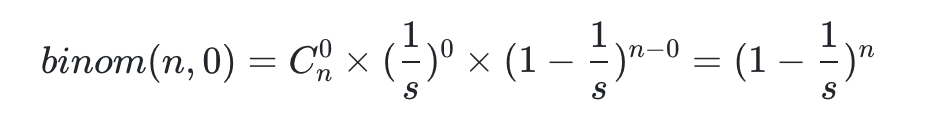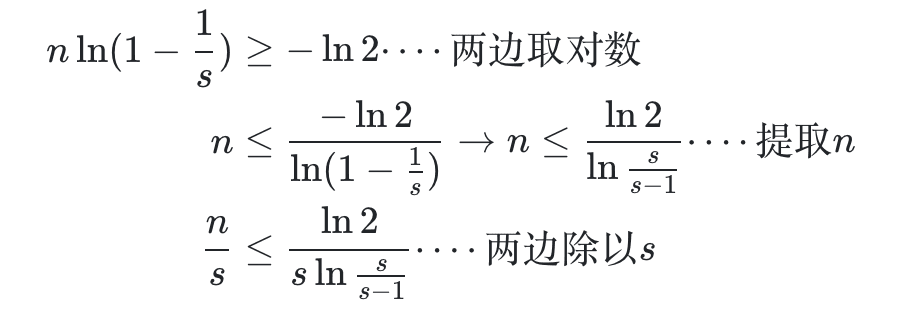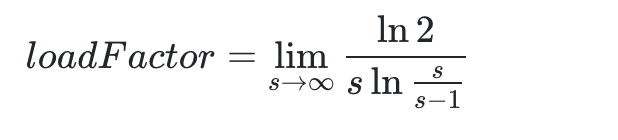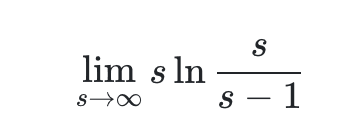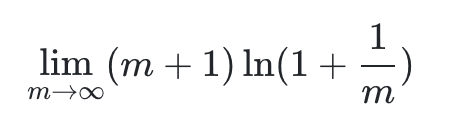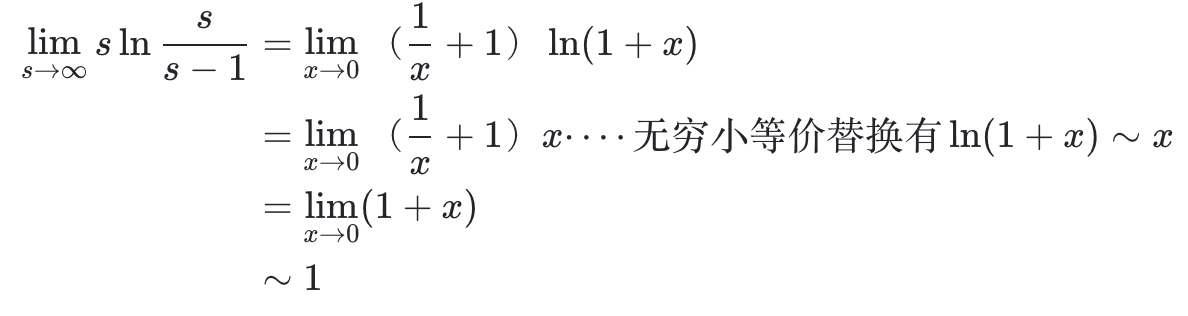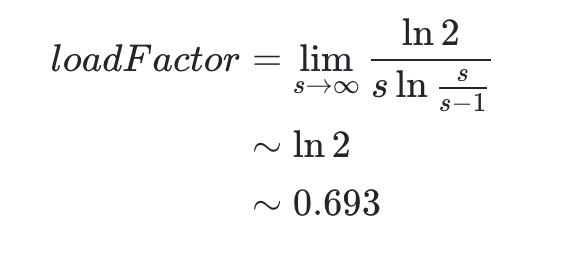16*0.75=12
32*0.75=24


## # 四、线程不安全

### # 01、多线程下扩容会死循环

JDK 7 时，采用的是头部插入的方式来存放链表的，也就是下一个冲突的键值对会放在上一个键值对的前面（同一位置上的新元素被放在链表的头部）。扩容的时候就有可能导致出现环形链表，造成死循环。

resize 方法的源码：

// newCapacity为新的容量
void resize(int newCapacity) {
// 小数组，临时过度下
Entry[] oldTable = table;
// 扩容前的容量
int oldCapacity = oldTable.length;
// MAXIMUM_CAPACITY 为最大容量，2 的 30 次方 = 1<<30
if (oldCapacity == MAXIMUM_CAPACITY) {
// 容量调整为 Integer 的最大值 0x7fffffff（十六进制）=2 的 31 次方-1
threshold = Integer.MAX_VALUE;
return;
}

// 初始化一个新的数组（大容量）
Entry[] newTable = new Entry[newCapacity];
// 把小数组的元素转移到大数组中
transfer(newTable, initHashSeedAsNeeded(newCapacity));
// 引用新的大数组
table = newTable;
// 重新计算阈值
threshold = (int)Math.min(newCapacity * loadFactor, MAXIMUM_CAPACITY + 1);
}


transfer 方法用来转移，将小数组的元素拷贝到新的数组中。

void transfer(Entry[] newTable, boolean rehash) {
// 新的容量
int newCapacity = newTable.length;
// 遍历小数组
for (Entry<K,V> e : table) {
while(null != e) {
// 拉链法，相同 key 上的不同值
Entry<K,V> next = e.next;
// 是否需要重新计算 hash
if (rehash) {
e.hash = null == e.key ? 0 : hash(e.key);
}
// 根据大数组的容量，和键的 hash 计算元素在数组中的下标
int i = indexFor(e.hash, newCapacity);

// 同一位置上的新元素被放在链表的头部
e.next = newTable[i];

// 放在新的数组上
newTable[i] = e;

// 链表上的下一个元素
e = next;
}
}
}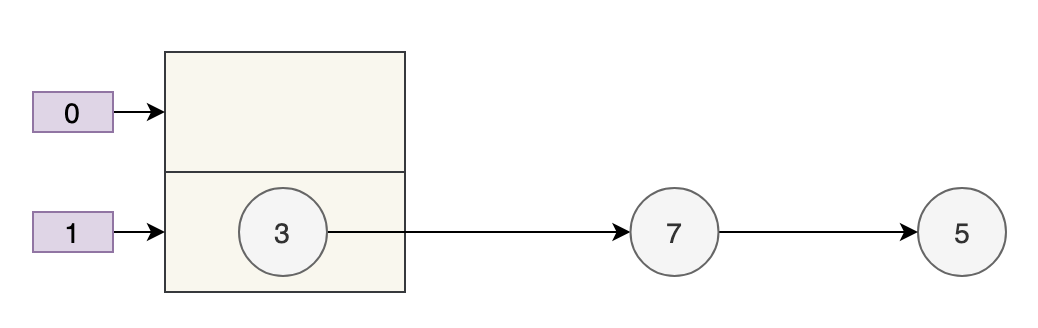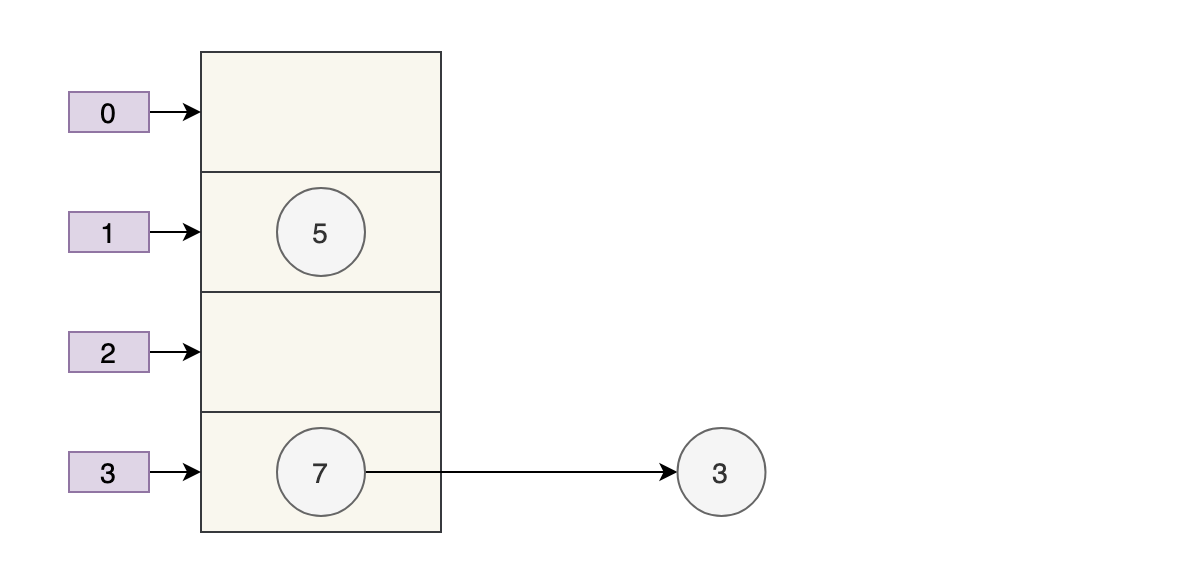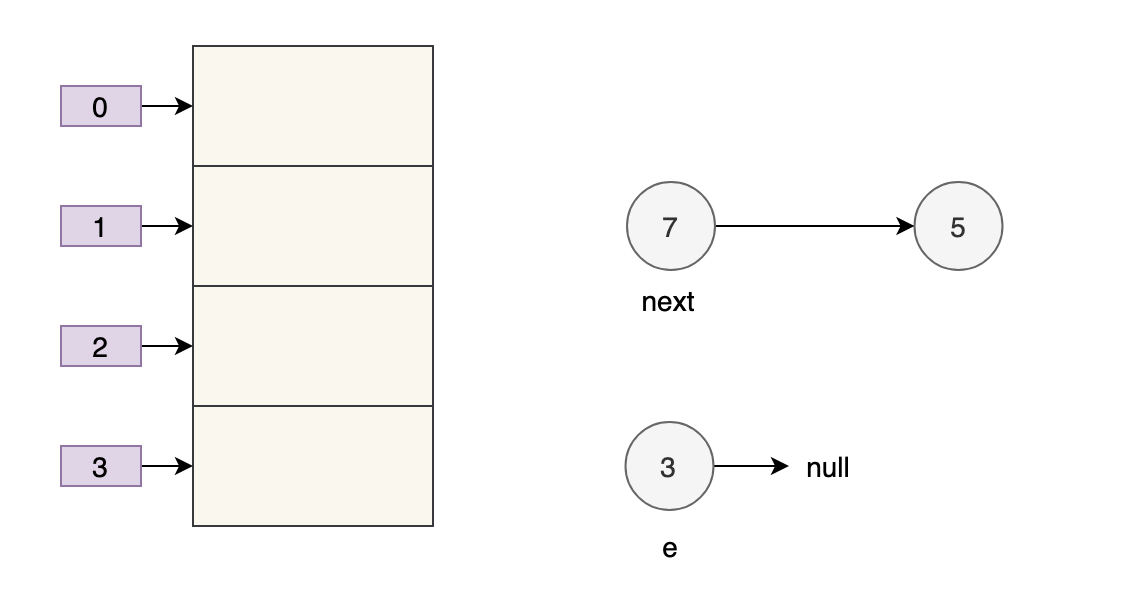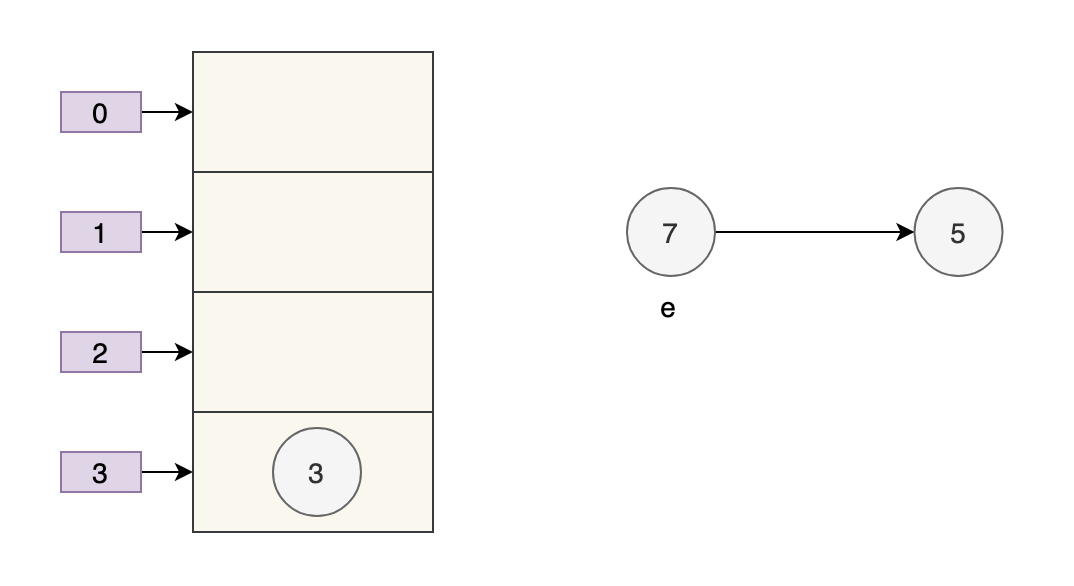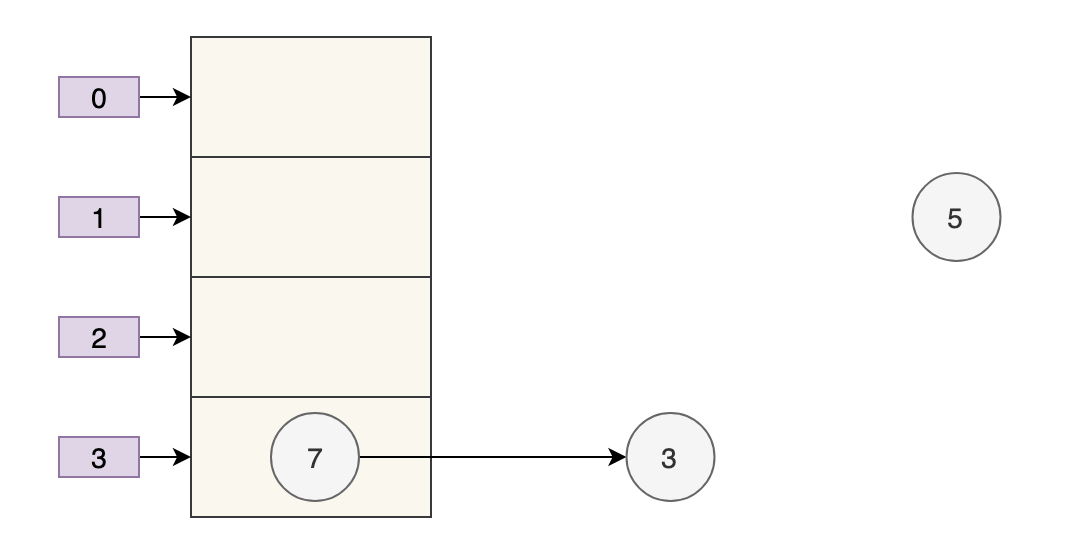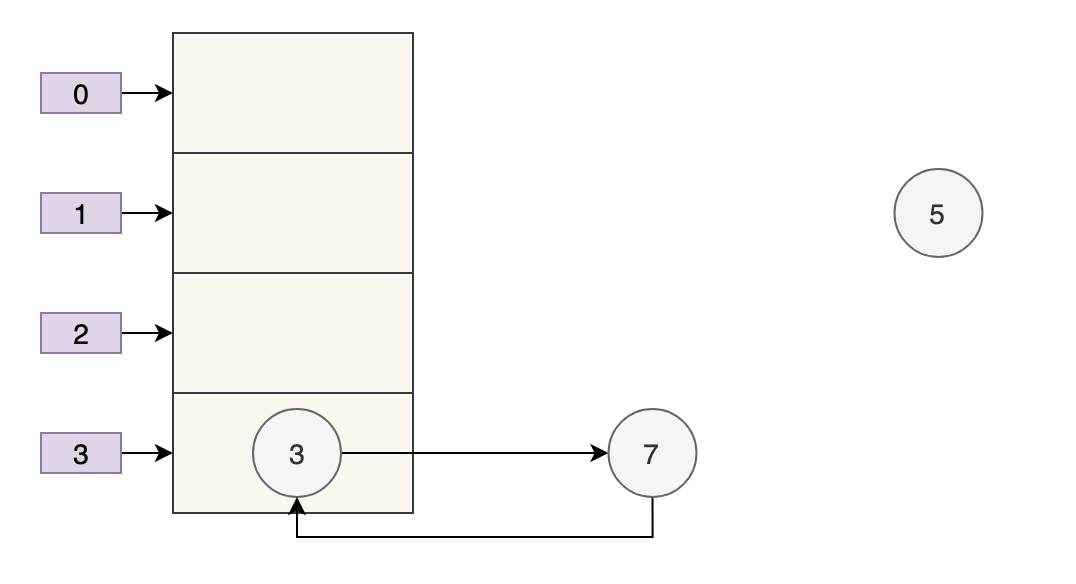### # 02、多线程下 put 会导致元素丢失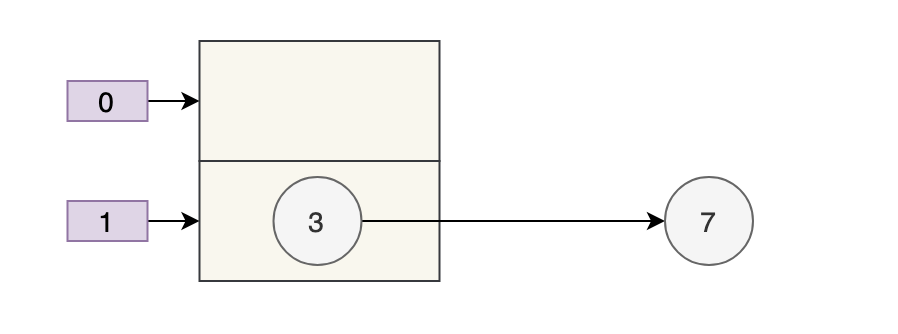put 的源码：

final V putVal(int hash, K key, V value, boolean onlyIfAbsent,
boolean evict) {
Node<K,V>[] tab; Node<K,V> p; int n, i;

// 步骤①：tab为空则创建
if ((tab = table) == null || (n = tab.length) == 0)
n = (tab = resize()).length;

// 步骤②：计算index，并对null做处理
if ((p = tab[i = (n - 1) & hash]) == null)
tab[i] = newNode(hash, key, value, null);
else {
Node<K,V> e; K k;

// 步骤③：节点key存在，直接覆盖value
if (p.hash == hash &&
((k = p.key) == key || (key != null && key.equals(k))))
e = p;

// 步骤④：判断该链为红黑树
else if (p instanceof TreeNode)
e = ((TreeNode<K,V>)p).putTreeVal(this, tab, hash, key, value);

// 步骤⑤：该链为链表
else {
for (int binCount = 0; ; ++binCount) {
if ((e = p.next) == null) {
p.next = newNode(hash, key, value, null);

//链表长度大于8转换为红黑树进行处理
if (binCount >= TREEIFY_THRESHOLD - 1) // -1 for 1st
treeifyBin(tab, hash);
break;
}

// key已经存在直接覆盖value
if (e.hash == hash &&
((k = e.key) == key || (key != null && key.equals(k))))
break;
p = e;
}
}

// 步骤⑥、直接覆盖
if (e != null) { // existing mapping for key
V oldValue = e.value;
if (!onlyIfAbsent || oldValue == null)
e.value = value;
afterNodeAccess(e);
return oldValue;
}
}
++modCount;

// 步骤⑦：超过最大容量 就扩容
if (++size > threshold)
resize();
afterNodeInsertion(evict);
return null;
}


if ((p = tab[i = (n - 1) & hash]) == null)
tab[i] = newNode(hash, key, value, null);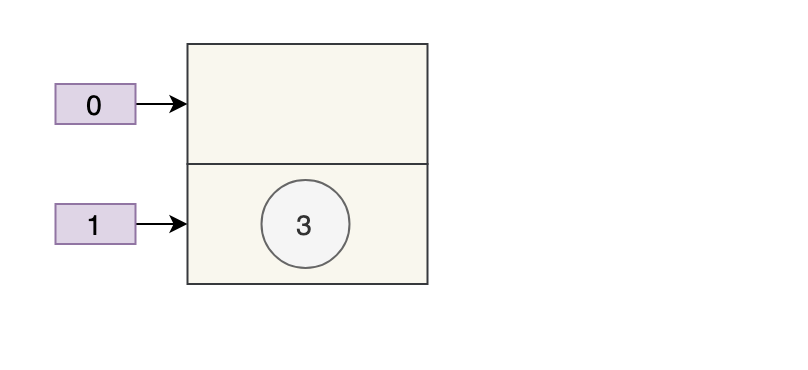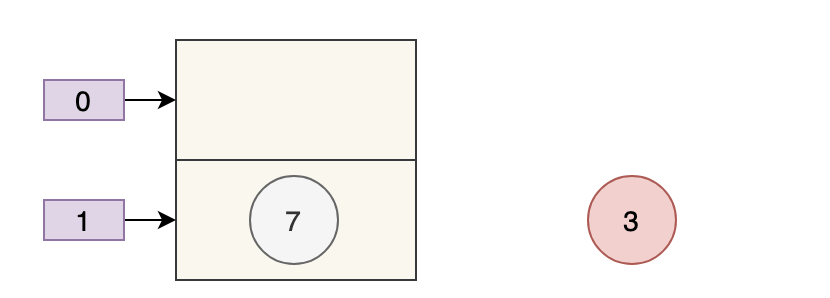3 被干掉了。

### # 03、put 和 get 并发时会导致 get 到 null

final Node<K,V>[] resize() {
Node<K,V>[] oldTab = table;
int oldCap = (oldTab == null) ? 0 : oldTab.length;
int oldThr = threshold;
int newCap, newThr = 0;
if (oldCap > 0) {
// 超过最大值就不再扩充了，就只好随你碰撞去吧
if (oldCap >= MAXIMUM_CAPACITY) {
threshold = Integer.MAX_VALUE;
return oldTab;
}
// 没超过最大值，就扩充为原来的2倍
else if ((newCap = oldCap << 1) < MAXIMUM_CAPACITY &&
oldCap >= DEFAULT_INITIAL_CAPACITY)
newThr = oldThr << 1; // double threshold
}
else if (oldThr > 0) // initial capacity was placed in threshold
newCap = oldThr;
else {               // zero initial threshold signifies using defaults
newCap = DEFAULT_INITIAL_CAPACITY;
}
// 计算新的resize上限
if (newThr == 0) {
float ft = (float)newCap * loadFactor;
newThr = (newCap < MAXIMUM_CAPACITY && ft < (float)MAXIMUM_CAPACITY ?
(int)ft : Integer.MAX_VALUE);
}
threshold = newThr;
@SuppressWarnings({"rawtypes","unchecked"})
Node<K,V>[] newTab = (Node<K,V>[])new Node[newCap];
table = newTab;
}